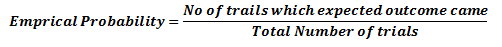Home/All Courses/CBSE/NCERT/NCERT Solutions For Class 9/NCERT Solution For Class 9 Maths/NCERT Solutions for Class 9 Maths Chapter 15 – Probability

## NCERT Solutions for Class 9 Maths Chapter 15 – Probability

Probability class 9 ncert solutions pdf covers the topic of Probability and probability formulas. To get in-depth knowledge on probability class 9 notes the students can refer to probability class 9 ncert solutions pdf given below.

## Probability

Probability is the measure of the likeliness that an event will occur. Probability is quantified as a number between 0 and 1 (where 0 indicates impossibility and 1 indicates certainty)

## Benefits of Probability

It is widely used in the study of Mathematics, Statistics, Gambling, Physical sciences, Biological sciences, Weather forecasting, Finance etc. to draw conclusions. Insurance companies use this to decide on financial policies.

## Terminology in Probabilty

### Randomness

In mathematics, When next outcome of the experiment cannot be determined then we say it is a random experiment.

### Trial

A trial is an action which results in one or several outcomes, for example each toss of the coin and each throw of the die are called trials.

### Independent Trial

Successive trials of some random event for example tosses of a coin, throws of a die are said to be independent if the outcome of any one trial does not impact the outcomes of any others.

### Experiment

Experiment and trial is same thing as such. An experiment is a situation involving chance or probability that leads to results called outcomes. But sometimes we use experiment to refer to whole large number of trials.

### Event

An event is a possible outcome of the Experiment. Like head coming in a toss

Sample space

It is a set of all possible outcomes of an experiment.

## Probability formulas

Few of the Probability formulas are:

P(A) = Number of favorable outcomes/ Total number of possible outcomes

## Empirical Probability

Experimental or empirical probability is an estimate that an event will happen based on how often the event occurs after performing an experiment in a large number of trials.

It is a probability of event which is calculated based on experimentsEmpirical probability depends on experiment and different will get different values based on the experiment.

2018-10-31T12:36:23+00:00 Categories: NCERT Solution For Class 9 Maths||0 Comments
Hello